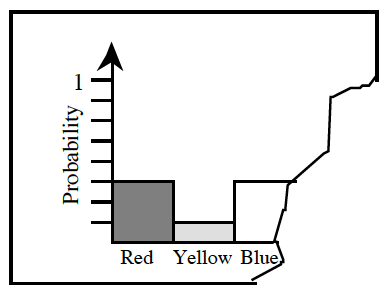### Home > GC > Chapter 5 > Lesson 5.1.3 > Problem5-28

5-28.

Donnell has a bar graph which shows the probability of a colored section coming up on a spinner, but part of the graph has been ripped off.1. What is the probability of spinning red?

How is the $y\text{-axis}$ on the graph scaled?

$\frac{3}{8}$

2. What is the probability of spinning yellow?

This problem is similar to part (a).

3. What is the probability of spinning blue?

Since you know the probabilities for red, yellow, and blue, and that all probabilities should add up to ____.
How can you find the probability for spinning green?

4. If there is only one color missing from the graph, namely green, what is the probability of spinning green? Why?